# Part B (Advanced) The figure below shows a long straight wire (Wire 1) carrying a constant current, I. A second wire (Wire 2), carrying a constant current, 12, is placed near the first wire with dime...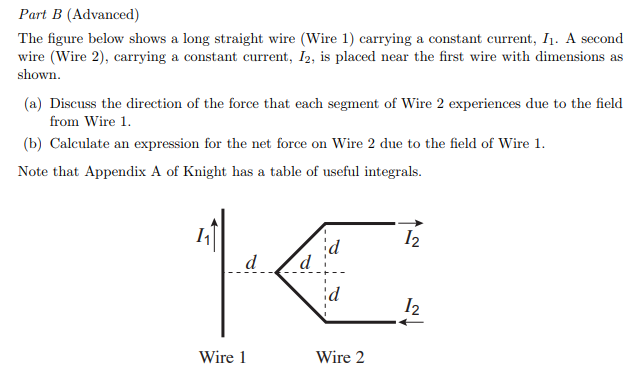Part B (Advanced) The figure below shows a long straight wire (Wire 1) carrying a constant current, I. A second wire (Wire 2), carrying a constant current, 12, is placed near the first wire with dimensions as shown (a) Discuss the direction of the force that each segment of Wire 2 experiences due to the field (b) Calculate an expression for the net force on Wire 2 due to the field of Wire 1. Note that Appendix A of Knight has a table of useful integrals. from Wire 12 12 Wire 1 Wire 2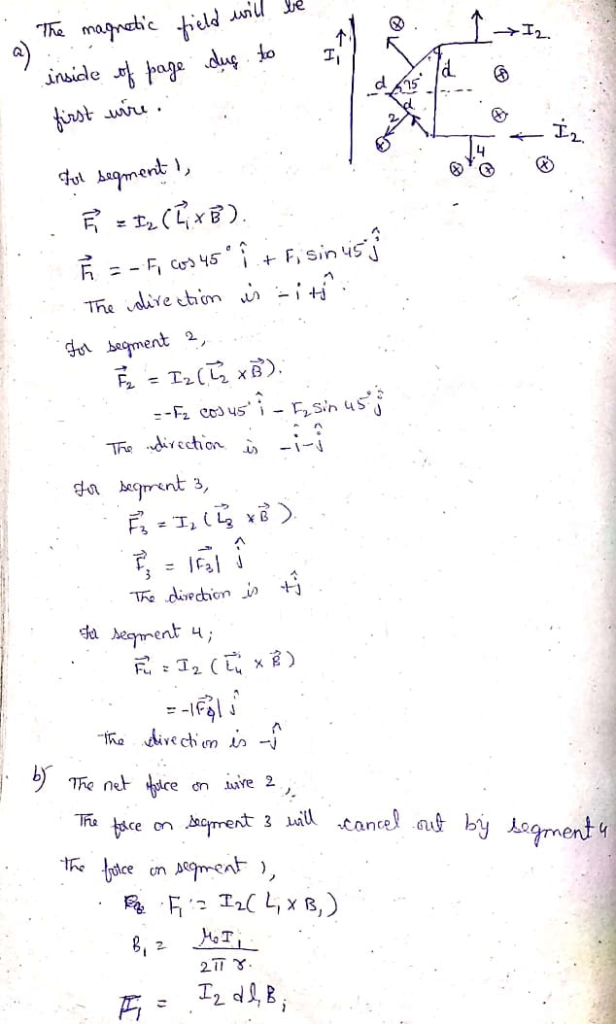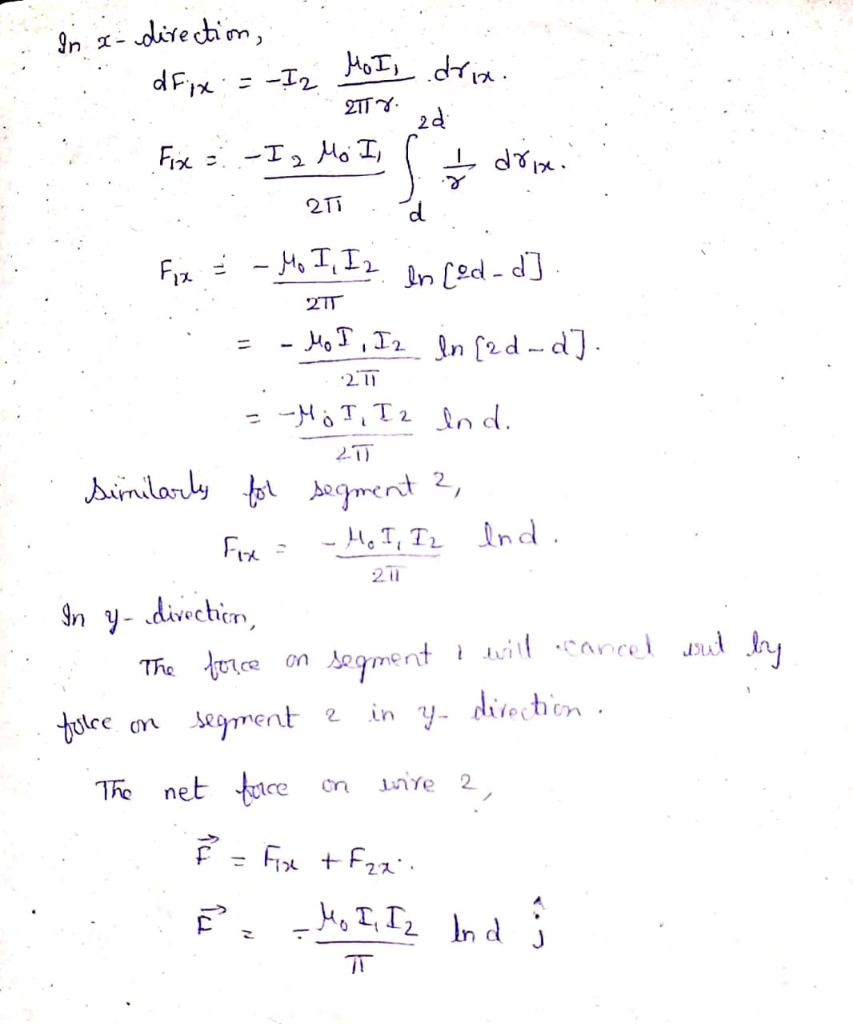##### Add Answer of: Part B (Advanced) The figure below shows a long straight wire (Wire 1) carrying a constant current, I. A second wire (Wire 2), carrying a constant current, 12, is placed near the first wire with dime...
Similar Homework Help Questions
• ### The figure below shows a long straight wire near a rectangular current loop. The current in...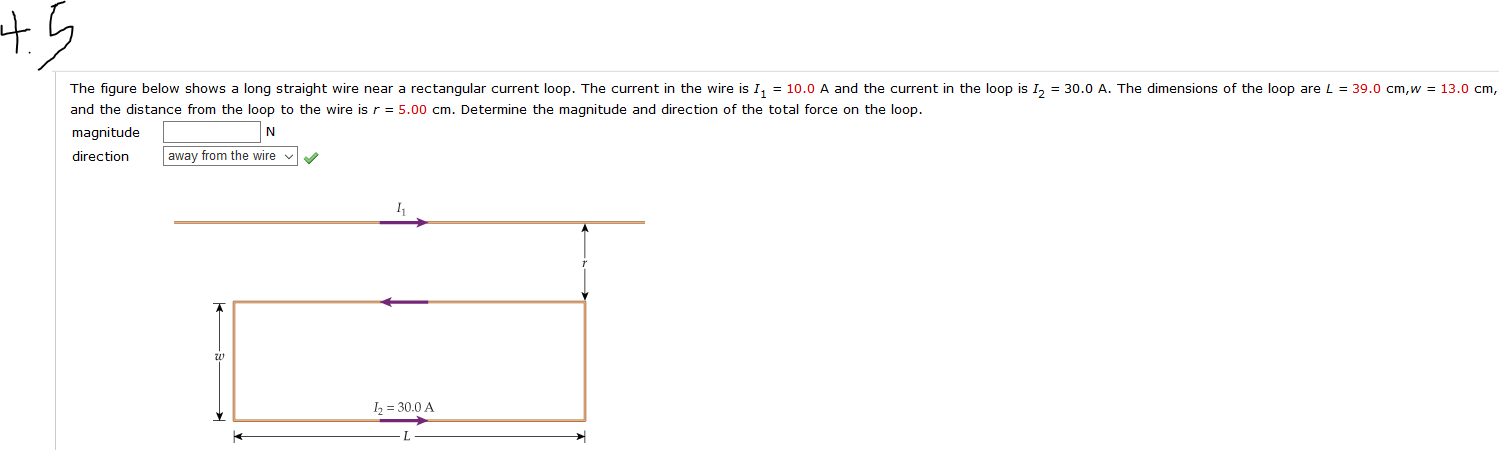The figure below shows a long straight wire near a rectangular current loop. The current in the wire is I = 10.0 A and the current in the loop is 1, = 30.0 A. The dimensions of the loop are L = 39.0 cm,w = 13.0 cm, and the distance from the loop to the wire is r = 5.00 cm. Determine the magnitude and direction of the total force on the loop. magnitude direction away from the wire 12...

• ### 1. The diagram below shows the deflection of a current carrying wire segment in a constant...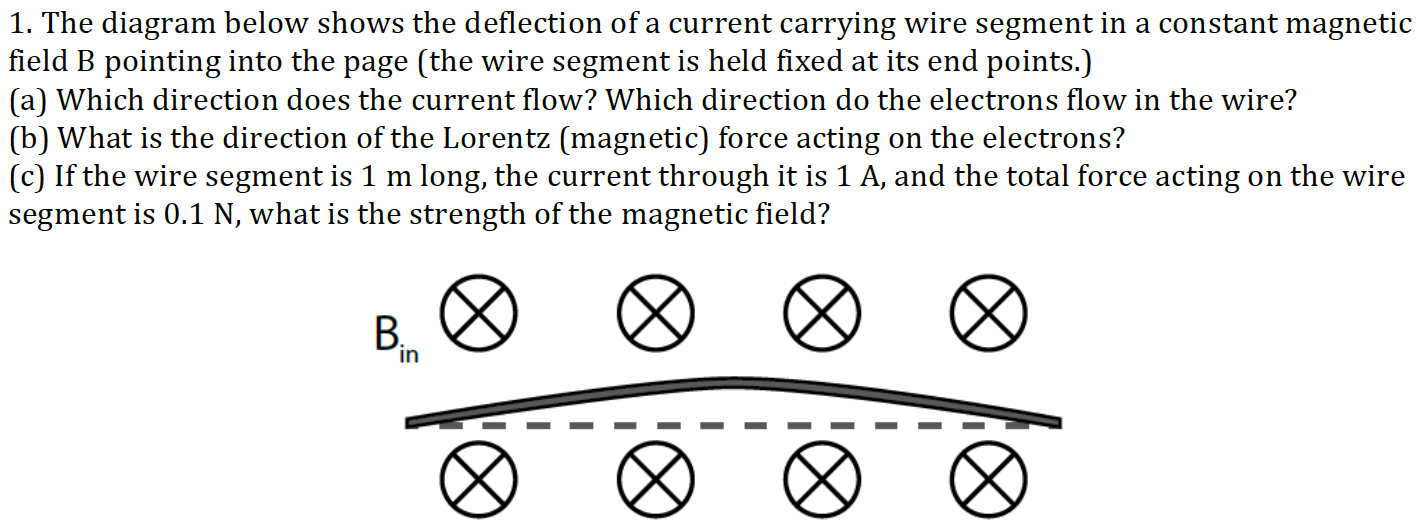1. The diagram below shows the deflection of a current carrying wire segment in a constant magnetic field B pointing into the page (the wire segment is held fixed at its end points.) (a) Which direction does the current flow? Which direction do the electrons flow in the wire? (b) What is the direction of the Lorentz (magnetic) force acting on the electrons? (c) If the wire segment is 1 m long, the current through it is 1 A, and...

• ### 4. (a) A long straight wire carrying a current is placed near a current-carrying rectangular loop...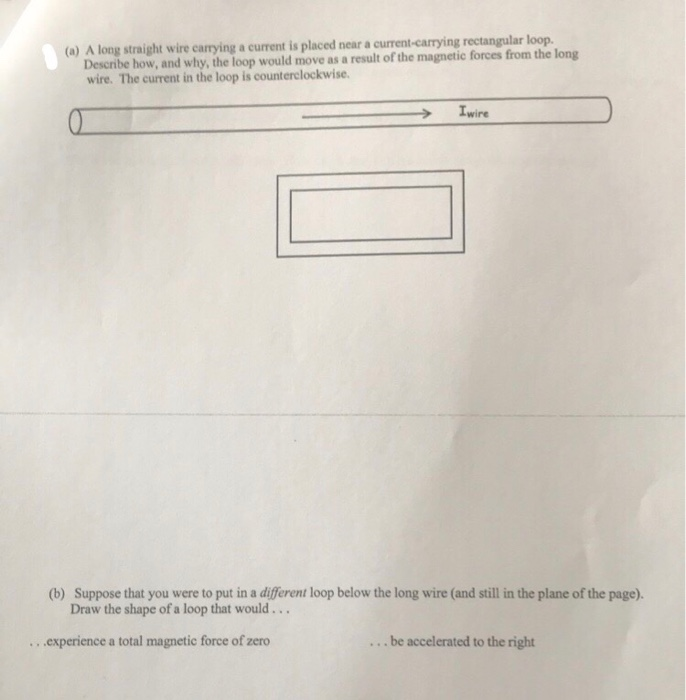4. (a) A long straight wire carrying a current is placed near a current-carrying rectangular loop. Describe how, and why, the loop would move as a result of the magnetic forces from the long wire. The current in the loop is counterclockwise. (b) Suppose that you were to put in a different loop below the long wire (and still in the plane of the page). Draw the shape of a loop that would... ...experience a total magnetic force of zero...

• ### A rectangular loop of wire is placed next to a straight wire. There is a current of 3.5A in both ...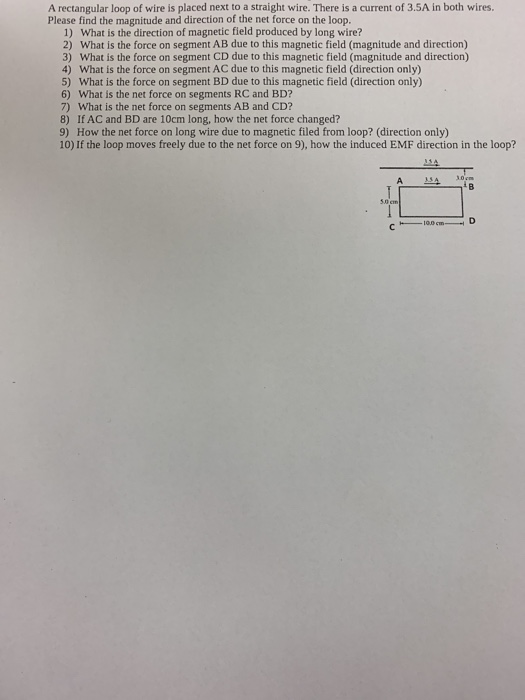A rectangular loop of wire is placed next to a straight wire. There is a current of 3.5A in both wires. Please find the magnitude and direction of the net force on the loop. 1) What is the direction of magnetic field produced by long wire? 2) What is the force on segment AB due to this magnetic field (magnitude and direction) 3) What is the force on segment CD due to this magnetic field (magnitude and direction) 4) What...

• ### 4) (12 pts) A long straight wire carrying a current I, is near a wire loop...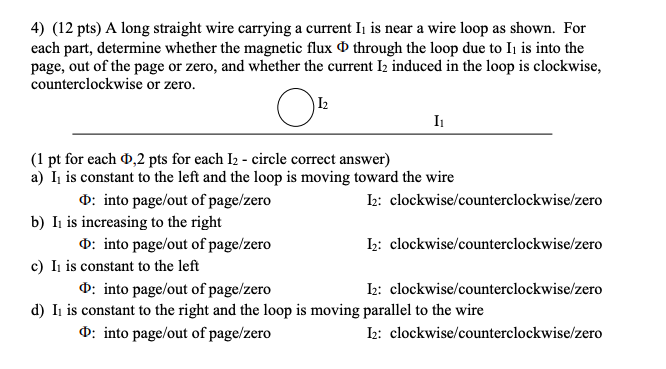4) (12 pts) A long straight wire carrying a current I, is near a wire loop as shown. For each part, determine whether the magnetic flux 0 through the loop due to Ii is into the page, out of the page or zero, and whether the current 12 induced in the loop is clockwise, counterclockwise or zero. 12 I O (1 pt for each 0,2 pts for each 12 - circle correct answer) a) I, is constant to the left...

• ### 1. The figure on the left shows the current, 1, of a long straight wire coming...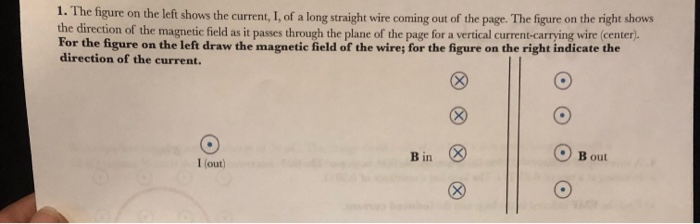1. The figure on the left shows the current, 1, of a long straight wire coming out of the page. The figure on the right shows the direction of the magnetic field as it passes through the plane of the page for a vertical current-carrying wire (center) For the figure on the left draw the magnetic field of the wire; for the figure on the right indicate the direction of the current. O O Bout I (out)

• ### In In the figure below, the current in the long, straight wire is 11 = 7.00...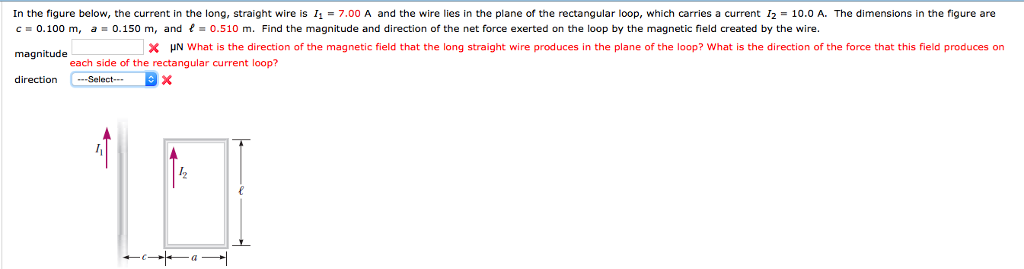In In the figure below, the current in the long, straight wire is 11 = 7.00 A and the wire lies in the plane of the rectangular loop, which carries a current 12 = 10.0 A. The dimensions in the figure are c = 0.100 m, a = 0.150 m, and f = 0.510 m. Find the magnitude and direction of the net force exerted on the loop by the magnetic field created by the wire. magnitude direction- Select-- μΝ...

• ### The diagram below shows a straight wire carrying a conventional current into the page. The wire...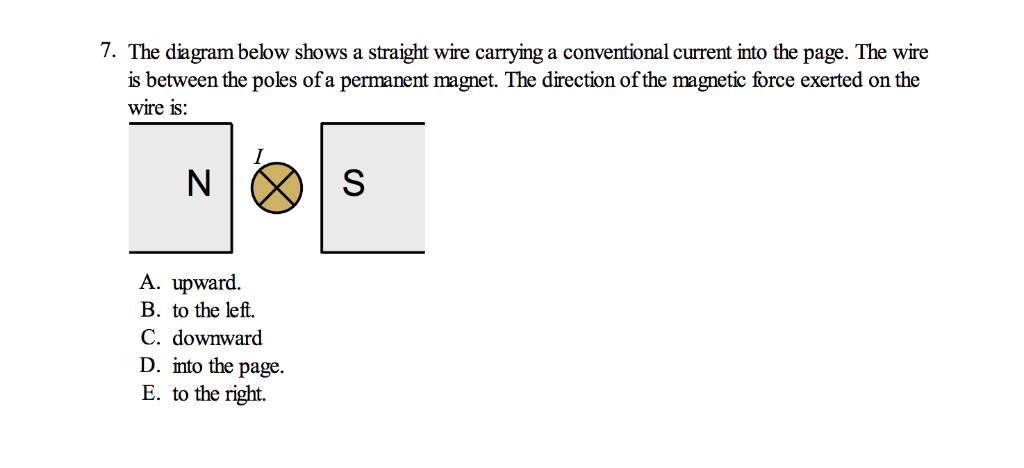The diagram below shows a straight wire carrying a conventional current into the page. The wire is between the poles of a permanent magnet. The direction of the magnetic force exerted on the wire is: A. upward. B. to the left. C. downward D. into the page. E. to the right. Answer key says C. downward; please explain. Thanks! 7. The diagram below shows a straight wire carrying a conventional current into the page. The wire is between the poles...

• ### A long straight wire carrying a 4-A current is placed along the x-axis as shown in the figure....A long straight wire carrying a 4-A current is placed along the x-axis as shown in the figure. What is the direction of the magnetic field at a pointP, located at y = 0.02 m, due to this wire?A) out of the plane of the pageB) along the +y-axisC) along the -x-axisD) along the +x-axisE )into the plane of the page

• ### The semicircular wire segment with radius a is carrying current I, and is placed in the...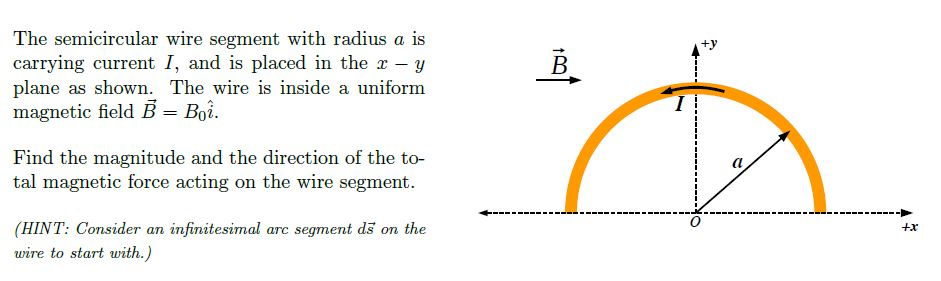The semicircular wire segment with radius a is carrying current I, and is placed in the x - y plane as shown. The wire is inside a uniform magnetic field B = Boi. Find the magnitude and the direction of the to- tal magnetic force acting on the wire segment. +x (HINT: Consider an infinitesimal arc segment ds on the wire to start with.)

Free Homework App